## Overview

In this guide, we describe how to set up Android Studio for native C++ development, and learn how to use Neon intrinsics for Arm-powered mobile devices.

Do not repeat yourself (DRY) is one of the major principles of software development. Following this principle typically means reusing your code using functions. However, invoking a function adds extra overhead. To reduce this overhead, compilers take advantage of built-in functions called intrinsics. The compiler replaces the intrinsics that are used in the high-level programming languages, for example C/C++, with mostly 1-1 mapped assembly instructions.

To further improve performance, you need assembly to use Assembly code. However, with Arm Neon intrinsics you can avoid the complication of writing assembly functions. Instead you only need to program in C/C++ and call the intrinsics or instruction functions that are declared in the arm_neon.h header file.

As an Android developer, you probably do not have time to write assembly language. Instead, your focus is on app usability, portability, design, data access, and tuning your app to various devices. If so, Neon intrinsics can help with performance.

Arm Neon intrinsics technology is an advanced Single Instruction Multiple Data (SIMD) architecture extension for Arm processors. SIMD performs the same operation on a sequence, or vector, of data during a single CPU cycle.

For instance, if you are summing numbers from two one-dimensional arrays, you must add them one by one. In a non-SIMD CPU, each array element is loaded from memory to CPU registers, the register values are added, and the result is stored in memory. This procedure is repeated for all elements. To speed up such operations, SIMD-enabled CPUs load several elements at once, perform the operations, then store results to memory. Performance improves depending on the sequence length, N. Theoretically, the computation time reduces N times.

Using SIMD architecture, Neon intrinsics can accelerate the performance of multimedia and signal processing applications, including video and audio encoding and decoding, 3D graphics, and speech and image processing. Neon intrinsics provide almost as much control as writing assembly code. However, Neon intrinsics leave the allocation of registers to the compiler. This allows developers to focus on the algorithms. Therefore, Neon intrinsics strike a balance between performance improvement and the writing of assembly language.

This guide shows you how to:

• Set up an Android development environment to use Neon intrinsics
• Implement an Android application that uses the Android Native Development Kit (NDK) to calculate the dot product of two vectors in C/C++
• Improve the performance of such a function with Neon intrinsics

At the end of this guide, you can Check your knowledge gained from this guide.

## Before you begin

This project was created with Android Studio. The sample code is available from the GitHub repository NeonIntrinsics-Android. The code was tested on a Samsung SM-J710F phone.

You should also explore the Neon intrinsics search engine.

## Native C++ Android project template

In this section we begin the development cycle by showing you what to select in your Android Studio options. Here are step by step instruction on what you need to do.

1. Create a project using the Native C++ Project Template, as you can see in the following screenshot: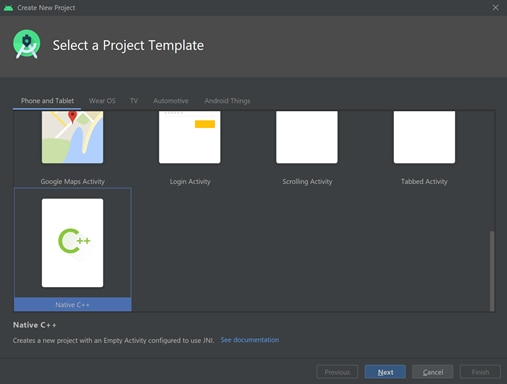2. Set the application name to Neon Intrinsics, selected Java as the language, and set the minimum SDK to API 19: Android 4.4 (KitKat) as shown in the follow screenshot: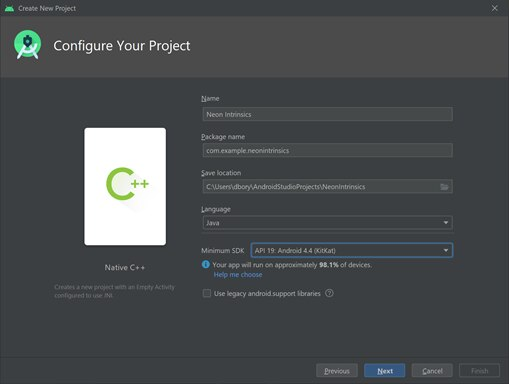3. Select Toolchain Default for the C++ Standard, as shown in the following screenshot: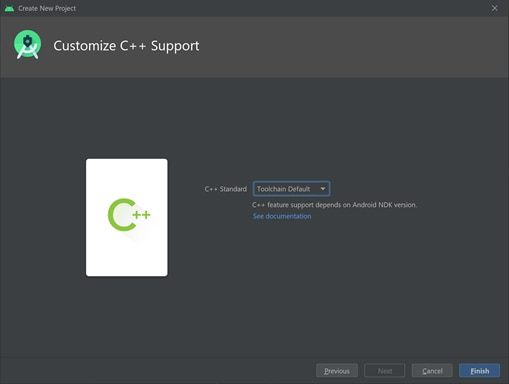The project that you created comprises one activity that is implemented within the `MainActivity` class, deriving from `AppCompatActivity` which you can see at app/java/com.example.neonintrinsics/MainActivity.java for further information. The associated view contains only a `TextView` control that displays the string: “Hello from C++, as you can see in the following image: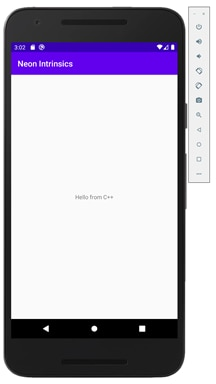To get these results, run the project directly from Android Studio using one of the emulators. To build the project successfully:

1. You must install `CMake` along with the Android NDK. You can do this through File > Settings. Then select NDK and CMake on the SDK Tools tab.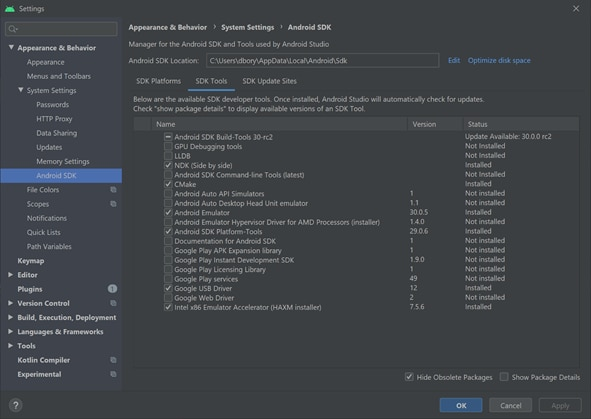2. Open the `MainActivity.java` file. The string that is displayed in the app comes from `native-lib`. The code for this library is in the `app/cpp/native-lib.cpp` file. That file is used for the implementation.

## Enabling Neon Intrinsics support

In this section we will look at enabling Neon Intrinsic support in Android Studio. To enable support for Neon intrinsics, we must modify the ABI filters & so the app can be built for the Arm architecture. There are two versions of Neon:

• Neon forArmv7, Armv8 AArch32
• Neon for Armv8 AArch64.

From an intrinsics point of view, there are a few differences, like the addition of vectors of 2xfloat64 in Armv8-A. Both versions are available in the arm_neon.h header file that is included in the compiler installation path. You also must import the Neon libraries.

1. Go to the `Gradle` scripts.
2. Open the `build.gradle` (Module: app) file.
3. Then, supplement the `defaultConfig` section by adding the following statements.
4. Add this line of code to the general settings:

`arguments "-DANDROID_ARM_NEON=ON"`

The code should look like this:

```defaultConfig {

applicationId "com.example.myapplication"

minSdkVersion 16

targetSdkVersion 29

versionCode 1

versionName "1.0"

ndk.abiFilters 'x86', 'armeabi-v7a', 'arm64-v8a'

testInstrumentationRunner "androidx.test.runner.AndroidJUnitRunner"

externalNativeBuild {

cmake {

cppFlags ""

arguments "-DANDROID_ARM_NEON=ON"

}

}

}```

Now you can use Neon intrinsics, which are declared within the `arm_neon.h` header. The build will only succeed for Arm-v7 and later. To make your code compatible with x86, use the Intel porting guide.

## Dot product and helper methods

In this section we look at dot product and helper methods. Now we can implement the dot product of two vectors using C++. All the code should be placed in the native-lib.cpp file. Starting from Armv8.4a, the dot product is part of the new instruction set. This corresponds to some Cortex-A75 designs and all Cortex-A76 designs onwards. Exploring the Arm dot product instructions includes for more information. Follow these steps:

• Beginning with the helper method that generates the ramp, which is the vector of 16-bit integers incremented from the code `startValue `in the following example:

```short* generateRamp(short startValue, short len) {
short* ramp = new short[len];
for(short i = 0; i < len; i++) {
ramp[i] = startValue + i;
}
return ramp;
}```
• Implement the `msElapsedTime` and now methods, which are used later to determine the code execution time:
```double msElapsedTime(chrono::system_clock::time_point start) {
auto end = chrono::system_clock::now();
return chrono::duration_cast<chrono::milliseconds>(end - start).count();
}

chrono::system_clock::time_point now() {
return chrono::system_clock::now();
}
</chrono::milliseconds>```

The msElapsedTime method calculates the amount of time, expressed in milliseconds, that has passed from a starting point. The now method is a handy wrapper for the `std::chrono::system_clock::now method`, which returns the current time.

• Create the actual dot product method. To calculate a dot product of two equal-length vectors, multiply vectors element-by-element, then accumulate the resulting products. Here you can see a straightforward implementation of this algorithm:
```int dotProduct(short* vector1, short* vector2, short len) {
int result = 0;

for(short i = 0; i < len; i++) {
result += vector1[i] * vector2[i];
}

return result;
}
```

The previous implementation uses a for loop. So we sequentially multiply vector elements and then accumulate the resulting products in a local variable called result.

## Calculating dot products using Neon Intrinsics

In this section of the guide, we look at calculating the dot products using Neon intrinsics. To modify the dotProduct function to benefit from Neon intrinsics, we must split the for loop so that it uses data lanes. This means that we will partition, or vectorize, the loop to operate on sequences of data during a single CPU cycle. These sequences are defined as vectors. However, to distinguish from the vectors that we use as inputs for the dot product, we call these sequences register vectors.

With register vectors, reduce the loop iterations so that, at every iteration, you multiply, then accumulate, multiple vector elements to calculate the dot product. The number of elements that you can work with depends on the register layout.

The Arm Neon architecture uses a 64-bit or 128-bit register file. In a 64-bit case, you can work with either eight 8-bit, four 16-bit, or two 32-bit elements. In a 128-bit case, you can work with either sixteen 8-bit, eight 16-bit, four 32-bit, or two 64-bit elements.

To represent various register vectors, Neon intrinsics use the following name convention:

In this convention:

`<type><size>x<number of lanes>_t`

In this convention:

• `<type>` is the data type (int, uint, float, or poly).
• `<size> `is the number of bits used for the data type (8, 16, 32, 64).
• ``` <number of lanes> defines how many lanes. ```

For example, int16x4_t represents a vector register with 4 lanes of 16-bit integer elements, which is equivalent to a four-element int16 one-dimensional array (short).

Do not instantiate Neon intrinsic types directly. Instead, use dedicated methods to load data from the arrays to CPU registers. The names of these methods start with vld. Method naming uses a convention that similar to the one for type naming. All methods start with v, which is followed by a method short name, like ld for load, and the combination of a letter and a number of bits, for example, s16, to specify the input data type.

Neon intrinsics directly correspond to the assembly instructions, as you can see in the following code:

```int dotProductNeon(short* vector1, short* vector2, short len) {
const short transferSize = 4;
short segments = len / transferSize;

// 4-element vector of zeros
int32x4_t partialSumsNeon = vdupq_n_s32(0);

// Main loop (note that loop index goes through segments)
for(short i = 0; i < segments; i++) {
// Load vector elements to registers
short offset = i * transferSize;
int16x4_t vector1Neon = vld1_s16(vector1 + offset);
int16x4_t vector2Neon = vld1_s16(vector2 + offset);

// Multiply and accumulate: partialSumsNeon += vector1Neon * vector2Neon
partialSumsNeon = vmlal_s16(partialSumsNeon, vector1Neon, vector2Neon);
}

// Store partial sums
int partialSums[transferSize];
vst1q_s32(partialSums, partialSumsNeon);

// Sum up partial sums
int result = 0;
for(short i = 0; i < transferSize; i++) {
result += partialSums[i];
}

return result;
}
```

To load data from memory, use the `vld1_s16` method. This method loads four elements to the CPU registers from the array of shorts signed 16-bit integers or `s16&`nbsp;for short,

When the elements are in the CPU registers, add the elements using the `vmlal` (multiply and accumulate) method. This method adds elements from two arrays and accumulates the result in a third array.

This array is stored within the `partialSumsNeon` variable. To initialize this variable, use the `vdupq_n_s32` (duplicate) method, which sets all CPU registers to the specific value. In this case, the value is 0. It is the vectorized equivalent of writing `int sum = 0.`

When all the loop iterations complete, store the resulting sums back to memory. The results can be read element by element `using vget_lane&`nbsp;methods. Alternatively, store the whole vector using `vst` methods. In this example, we use the second option.

When the partial sums are back in memory, I sum them to get the final result.

On AArch64, you could also use:

`return vaddv_s32 (partialSumsNeon);`

Then skip the second for loop.

## Putting our code together

We can now put all of the code together. To do this, we modify the `MainActivity.stringFromJNI` method in the following code:

```extern "C" JNIEXPORT jstring JNICALL
MainActivity.stringFromJNI (
JNIEnv* env,
jobject /* this */) {

// Ramp length and number of trials
const int rampLength = 1024;
const int trials = 10000;

// Generate two input vectors
// (0, 1, ..., rampLength - 1)
// (100, 101, ..., 100 + rampLength-1)
auto ramp1 = generateRamp(0, rampLength);
auto ramp2 = generateRamp(100, rampLength);

// Without NEON intrinsics
// Invoke dotProduct and measure performance
int lastResult = 0;

auto start = now();
for(int i = 0; i < trials; i++) {
lastResult = dotProduct(ramp1, ramp2, rampLength);
}
auto elapsedTime = msElapsedTime(start);

// With NEON intrinsics
// Invoke dotProductNeon and measure performance
int lastResultNeon = 0;

start = now();
for(int i = 0; i < trials; i++) {
lastResultNeon = dotProductNeon(ramp1, ramp2, rampLength);
}
auto elapsedTimeNeon = msElapsedTime(start);

// Clean up
delete ramp1, ramp2;

// Display results
std::string resultsString =
"----==== NO NEON ====----\nResult: " + to_string(lastResult)
+ "\nElapsed time: " + to_string((int)elapsedTime) + " ms"
+ "\n\n----==== NEON ====----\n"
+ "Result: " + to_string(lastResultNeon)
+ "\nElapsed time: " + to_string((int)elapsedTimeNeon) + " ms";

return env->NewStringUTF(resultsString.c_str());
}
```

The `MainActivity.stringFromJNI` method proceeds as follows:

1. Create two equal-length vectors using `generateRamp` methods.
2. Calculate the dot product of those vectors using the non-Neon method `dotProduct`. Repeat this calculation several times (trials constant) and measure the computation time using `msElasedTime`.
3. Perform the same operations as in Step 1 and Step 2, but now using the Neon-enabled method `dotProductNeon.`
4. ` `Combine the results of those two methods along with the computation times within the `resultsString`. The latter is displayed in the `TextView`. To build and run the preceding code successfully, you need an Arm-v7-A or Armv8-A device. The following image shows the improvements that Neon Intrinsics can bring to an application: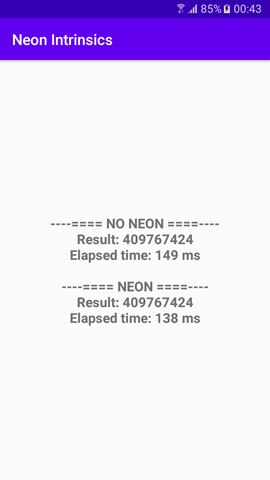Using built-in intrinsics provided a seven percent improvement in elapsed time. A theoretical improvement of 25 percent could be achieved on Arm 64 devices.

Q: What are the benefits of using Neon Intrinsics compared to using assembly code?

A: Compilers take advantage of built-in functions called intrinsics, with mostly 1-2-1 mapped assembly instructions. Using intrinsics removes the need to use Assembly code to get the highest performance out of the underlying hardware.

Q: What are some example areas that can be improved by using SIMD architecture?

A: Some examples include video and audio encoding and decoding, 3D graphics, and speech and image processing.

Q: What are the name conventions that Neon intrinsics use to represent various register vectors?

A: To represent various register vectors, Neon intrinsics use the following name convention:

`<type><size>x<number of lanes>_t`

• `<type>` is the data type (int, uint, float, or poly).
• `<size> `is the number of bits used for the data type (8, 16, 32, 64).
• ``` <number of lanes> defines how many lanes. ```

## Related information

Here are some resources related to material in this guide:

Arm Resources

Android Resources

Other Resources

## Next Steps

This guide introduced the fundamental principles of using Neon intrinsics with an Android-based device. In the guide, we saw how to set up Android Studio for native C++ development, and how to use Neon intrinsics for Arm-powered mobile devices.

After explaining the idea behind Neon intrinsics, we demonstrated a sample implementation of the dot product of two equal-length vectors. We then vectorized the method using dedicated Neon intrinsics. In particular, we described loading data from memory to CPU registers, completing the operations, and then storing the results back to memory.

Vectorizing code is never an easy task. However, you can simplify it with Neon intrinsics to improve performance in scenarios that employ 3D graphics. An example of this would be signal and image processing, audio encoding, and video streaming.

Now you can start using the Neon intrinsics in your own projects. If you want to learn more about Neon, you can read our guide Neon Intrinsics on Android: How to Truncate Thresholding and Convolution of a 1D Signal.